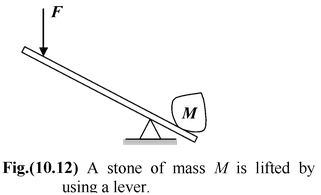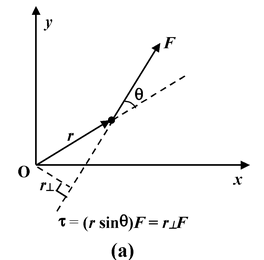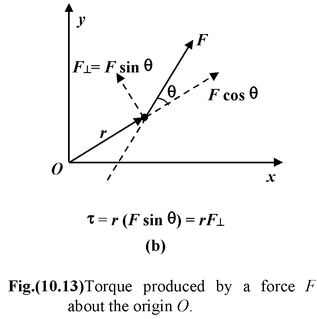# Torque

## Rotational Motion of Class 11

 Torque is the rotational analog of force. Force causes linear acceleration; torque causes angular acceleration. In order to lift a stone by using a lever, a force is required (see figure 10.12). The effectiveness of the force depends both on its direction and where it is applied relative to the pivot point.The turning ability of a force about an axis or pivot is called its torque. The torque of a force F that acts at a distance r from the origin is defined as  T = rF sinθ (10.20) Where θ is the angle between the vector r and F, as shown in the figure (10.13).The definition of torque (equation 10.20) may be interpreted in two ways: (i) T = r (Fsinθ) = r  ⊥ F The turning effect of a force about the origin is produced only by the perpendicular component (F⊥ = F sin θ) as shown in the figure (10.8 b). (ii) T = (r sinθ) F = r⊥ F Where r⊥ is the perpendicular distance from the origin to the line of action of the force as shown in the figure (10.8 a). It is also called the lever arm.